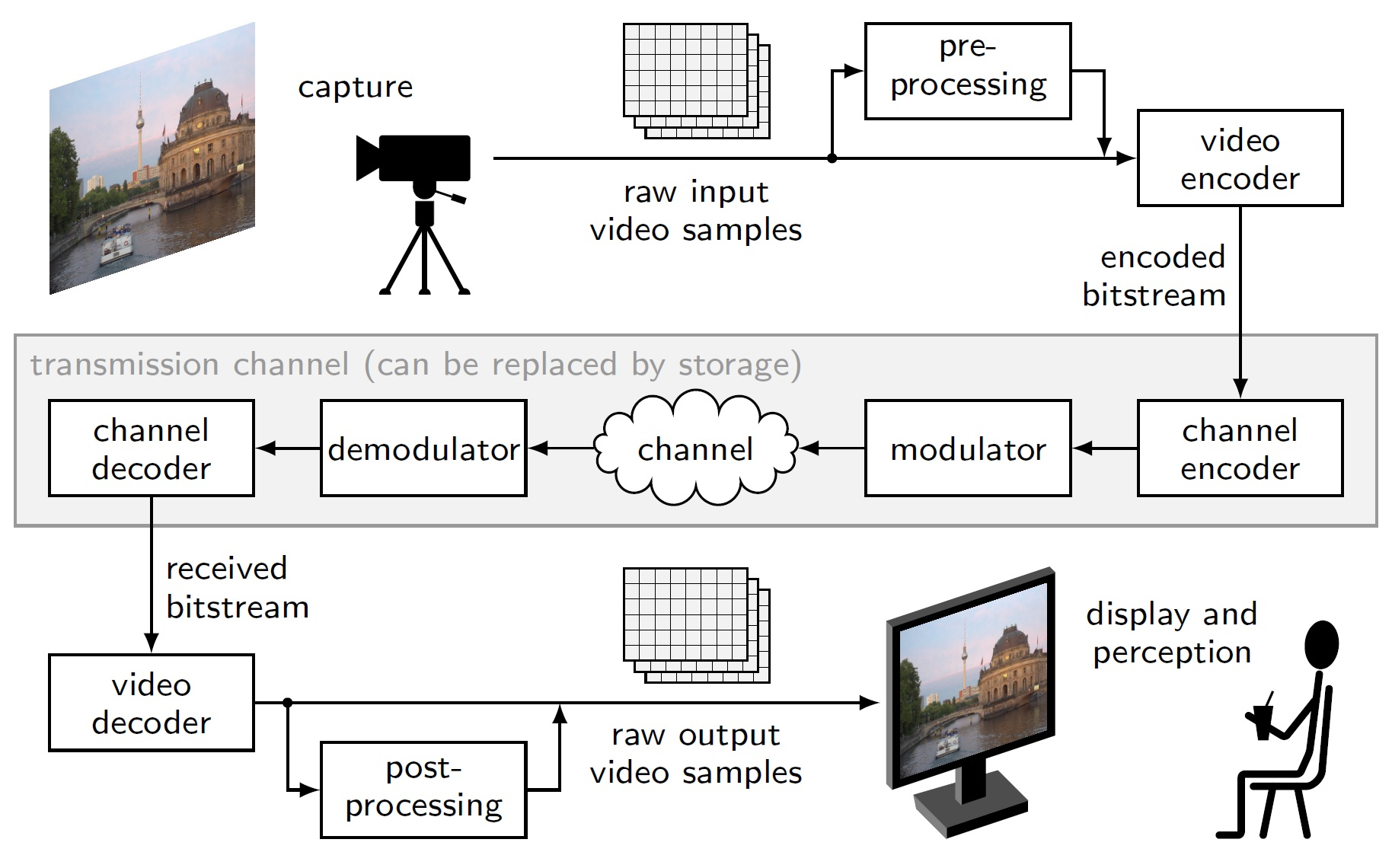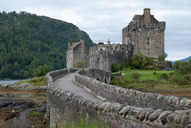Fraunhofer Heinrich Hertz Institute
Prof. Dr.-Ing. Heiko Schwarz
Image and Video Coding I: FundamentalsExercises

• [Oct 22] Exercises - Set A (pdf)

• [Oct 29] Exercises - Set B (pdf)

• [Nov 05] Exercises - Set C (pdf)

• [Nov 12] Exercises - Set D (pdf)

• [Nov 19/26] Exercises - Set E (pdf, framework, Benjamin's code, Musterlösung)

• [Dec 3] Exercises - Set F (pdf)

• [Dec 10] Exercises - Set G (pdf)

• [Dec 17] Exercises - Set H (pdf)

• [Jan 07] Exercises - Set I (pdf)

• [Jan 14] Exercises - Set J (pdf)

• [Jan 21] Exercises - Set K (pdf)

• [Jan 28] Exercises - Set L (pdf)

• [Feb 04] Exercises - Set M (pdf)

• [Feb 11] Exercises - Set N (pdf)

• Lecture Slides

Introduction to Source, Image and Video Coding
• Introduction (pdf)

Lossless Coding
• Introduction, Discrete Random Processes (pdf)

• Prefix codes, Unique decodability, Entropy, Huffman algorithm (pdf)

• Conditional codes, Block codes, V2V codes, Entropy rate, Shannon-Fano-Elias codes (pdf)

• Iterative Shannon-Fano-Elias coding, Arithmetic coding, Summary on Lossless Coding (pdf)

Rate-Distortion Theory
• Introduction, Continuous Random Processes (pdf)

• Mutual Information, Information Rate-Distortion Function, Differential Entropy (pdf)

• Diff. Entropy Rate for Gaussians, Shannon Lower Bound, Rate-Distortion Function for MSE and Gaussians (pdf)

Quantization
• Introduction, Scalar Quantization: Centroid Quantizer, Lloyd Quantizer (pdf)

• Scalar Quantization: Entropy-Constrained Lloyd Quantizer (pdf)

• Uniform Reconstruction Quantizer; Vector Quantization (pdf)

Transform Coding
• Overview, Unitary and Orthogonal Block Transforms, Optimal Bit Allocation for Transform Coefficients (pdf)

• The Karhunen–Loève Transform (KLT), Optimality of the KLT, High-Rate Approximations for Gaussians (pdf)

• Signal Independent Transforms, DCT-II, Transform Coding in Practice (pdf)

Predictive Coding
• Affine and Linear Prediction (pdf)

• Combination of prediction and quantization (pdf)

Preview of part II and Notes on Exam
• Preview: Image and Video Coding II: Algorithms and Applications (pdf)

• Notes on Exam (pdf)

Raw image files in PPM formatLiteratur
• T. M. Cover and J. A. Thomas: Elements of Information Theory,
John Wiley & Sons, New York, 2006.

• A. Gersho and R. M. Gray: Vector Quantization and Signal Compression,
Kluwer Academic Publishers, Boston, Dordrecht, London, 1992.

• N. S. Jayant and P. Noll: Digital Coding of Waveforms,
Prentice-Hall, Englewood Cliffs, NJ, 1994.

• Thomas Wiegand and Heiko Schwarz:
Source Coding: Part I of Fundamentals of Source and Video Coding,
Foundations and Trends in Signal Processing, vol. 4, no. 1-2, pp. 1-222, January 2011.